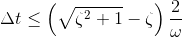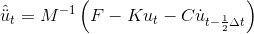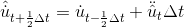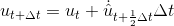# Explicit Difference

This command is used to construct a Explicit Difference integrator object.

 integrator Explicitdifference

EXAMPLE:

integrator Explicitdifference

NOTES:

1. When using Rayleigh damping, the damping ratio of high vibration modes is overrated, and the critical time step size will be much smaller. Hence Modal damping is more suitable for this method.
2. There should be no zero element on the diagonal of the mass matrix when using this method.
3. Diagonal solver should be used when lumped mass matrix is used because the equations are uncoupled.
4. For stability,THEORY:

The Explicit Difference Integrator is based on the Leap-frog method. The basic formula of Leap-frog method is shown as below:As mass matrix M is a diagonal matrix, the equations can be uncoupled, then the solution procedure will be very efficient.

Code Developed by: Shuhao Zhang, Tsinghua University, and Prof. Xinzheng Lu, Tsinghua University